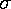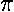## Descriptive Statistics

They are different, but not different enough to matter -- like the maple leaves off the tree in my yard, when all I want to do is rake them up.

Roald Hoffmann, 1981 Nobel Laureate in Chemistry
from: The Same and Not the Same

If you were to measure the size of 10 maple leaves you would quickly find that maple leaves in fact come in different sizes. Thus it is impossible to report the size of maple leaves, instead the best you can do is to report a typical size and give some estimate of the range of variation above and below that typical size. The attempt to capture the full meaning of "the size of maple leaves" in a few numbers is bound to fail -- Nature really is more complex than our descriptions of it. Nevertheless if our choice is to be silent on "the size of maple leaves" or to provide a list of the size of every maple leaf in the world (on this day) or to provide a few summarizing numbers, the latter is the option selected by science. This page introduces a handful of statistics which are commonly used to describe the distribution of data.

### Typical Values

There are several common methods of selecting a "typical" value for data. The most common method is the average or mean. To obtain an average value, add up all your data values and divide by the number of data items. If X01 is the length of your first maple leave, X02 the length of your second maple leave, etc., then the average maple leaf length is:

(X01+X02+X03+ X04+X05+X06+ X07+X08+X09+ X10)/10 = Xavg

To obtain the median value, first sort your list of leaf-lengths from lowest to highest:

{5.1, 7.2, 4.1, 9.5, 6.7, 7.8, 8.5, 7.0, 7.3, 9.0} becomes:

{4.1, 5.1, 6.7, 7.0, 7.2, 7.3, 7.8, 8.5, 9.0, 9.5}

and then select the value in the exact middle as the median. (It turns out that if the number of items is even, as in this example, there is no exact middle. 7.2 is 5 places from the front and 6 places from the back; 7.3 is 6 places from the front and 5 places from the back. So with even-numbered data sets, average the two near-middle values, producing Xmed=7.25 in this example.)

The mode "typical" value will be of less use to us: it is the most repeated value in the data set. In the above example, no value is repeated (each value occurs exactly once). This is commonly the case with so few data items; hence its limited utility for us.

The geometric mean is useful for "log-normal distributions". To obtain the geometric mean, multiply all the numbers together and then take the result to the power 1/N (where N is the number of data items -- 10 in our example). So the geometric mean is:

(X01·X02·X03· X04·X05·X06· X07·X08·X09· X10)1/10 = Xgeo

### Estimates of the Range of Variation

In some sense, the range of variation is limited only by your willingness to search through ever larger piles of leaves. Generally, the more data you record the more extreme your highs and lows will be. Nevertheless, you should find that the range of leaf lengths, that includes say 50% of your sample, remains about the same even if you look through ever larger piles of leaves. That is to say, there is a common range of variation even as larger data sets produce rare "outliers" with ever more extreme deviation. Estimates of the range of variation seek to put a number to this common range of variation that doesn't depend on sample size.

The most common way to describe the range of variation is standard deviation (usually denoted by the Greek letter sigma:). The standard deviation is simply the square root of the variance, so lets start by describing the variance. To obtain the variance start by subtracting the average from each data item. Since there will be about as many items above average as below average, the resulting list of numbers will have about as many positive values as negative values. (In fact this list of deviations-from-average must itself average to zero!) Square each deviation, and proceed to find the average of the squared-deviations. However, in finding the average squared-deviation, divide by N-1 rather than N. The result is the variance; take its square root to get the standard deviation.

variance = ( (X01-Xavg)2 + (X02-Xavg)2 + (X03-Xavg)2 + ··· + (X10-Xavg)2 )/9

For data that is "normally distributed" we expect that about 68.3% of the data will be within 1 standard deviation of the mean (i.e., in the range Xavg ±). In general there is a relationship between the fraction of the included data and the deviation from the mean in terms of standard deviations.

```Fraction    Number of Standard
of Data    Deviations from Mean

50.0%           .674
68.3           1.000
90.0           1.645
95.0           1.960
95.4           2.000
98.0           2.326
99.0           2.576
99.7           3.000```
Thus we should expect that 95% of the data would be within 1.96 standard deviations of the mean (i.e., in the range Xavg ± 1.96). This is called a 95% confidence interval for the sample.

The average deviation or mean absolute deviation is calculated in a similar manner as standard deviation, except here you subtract the median from each data item producing a list of deviations from the median. Instead of squaring each deviation, you take the absolute value of each deviation. Finally you average in the usual way: using N not N-1.

average deviation = ( |X01-Xmed | + |X02-Xmed | + |X03-Xmed | + ··· + |X10-Xmed | )/10

If the data is "normally distributed" there is a definite relationship between the average deviation and the standard deviation:

average deviation = 0.80 × standard deviation;
where 0.80 = (2/)½.

### Five-Number Summary

The median, you recall, is in the middle of the sorted data. In a similar way we can define the first quartile to be 1/4 of the way through the sorted data, and the third quartile to be 3/4 of the way through the sorted data. (The preferred name for the second quartile is the median, so that's what we'll call it.) The range between the first and third quartiles includes half of the data. The size of the range (i.e., the difference between the third quartile and the first quartile) is another measurement of variability called the interquartile range or IQR. For normally distributed data:

IQR = 1.35 ×The five-number summary of the data consists of the minimum data point, the first quartile, the median, the third quartile, and the maximum data point. Thus these five numbers display the full range of variation (from minimum to maximum), the common range of variation (from first to third quartile), and a typical value (the median).

### Standard Deviation of the Estimated Means

The above procedure describes how to define a "typical" leaf using 10 sample leaves. Clearly if another group uses the same procedure on its own sample of 10 leaves, it is unlikely to come up with exactly the same value for a "typical" leaf. How much variation is there in the estimates of "typical" described above? Clearly if we expand the sample beyond 10 (to 100, or 1000, ...) we would expect to come closer to the actual "typical" leaf (i.e., that determined by looking at all the leaves in the world). Thus the larger the sample you average over, the smaller is your expected deviation from the exact result. But how much variation should you expect in a calculated average leaf? The standard deviation expected in a calculated average is:/N1/2

Thus the deviations expected equal the standard deviation of the length of leaves if you "average" over just one leaf, and decrease as the square root of N as N increases. Thus one can expect to get quite close to the exact mean if the sample size N gets very big.

### "Normal" and other Distributions

Many pages have been written by others on this topic. To be brief, a common assumption of statistics-users is that data is "normally" distributed. Occasionally the folks making this assumption know what it means and even test to see if it's a valid assumption. I'm going to leave you in the dark (like many statistics-users) about what this assumption means and how you test it. There are several good courses and books that would include these topics. I will give you two (not very helpful) hints.
1. (Bad News) Many things in nature are not "normally" distributed. (Good News) Much of what is not "normally" distributed in biology would be "normally" distributed if you took the logarithm of each data item. Thus there is a button on the descriptive statistics calculation page to do this conversion for you. The result is that the geometric mean is calculated for you and a different kind of standard deviation is produced. With the usual standard deviation you add or subtract the standard deviation from the mean in order to test for fractions of included data; with the log standard deviation, you multiply or divide. Thus you would expect 68.3% of your data to be between Xgeo×and Xgeo÷; 95.4% of your data would be between Xgeo×2 and Xgeo÷2
2. (Bad News) Much of what's in books about statistics has to do with "normally" distributed data. Statistics that provide useful information even if applied to not-"normally" distributed data are call robust statistics. Median and average deviation are considered robust statistics. (Good News) The program always calculates them for you.

There is one additional distribution you should know a bit about: the Poisson distribution. The Poisson distribution particularly applies to counts of things, like the number of maple trees per acre or the number of radioactive decays in an hour. The main upshot is that with things distributed according to the Poisson distribution, the standard deviation of the count can be estimated as the square root of the count. Thus if in a particular experiment you detected 1000 radioactive decays, a full repeat of that experiment might yield 1030 or 970 or 1037 or 998... The standard deviation of the counts in the repeated experiments should be close to the square root of 1000 (31.6). Thus if you don't have the time to do lots of full repeats, the range of variation you would experience can still be estimated.